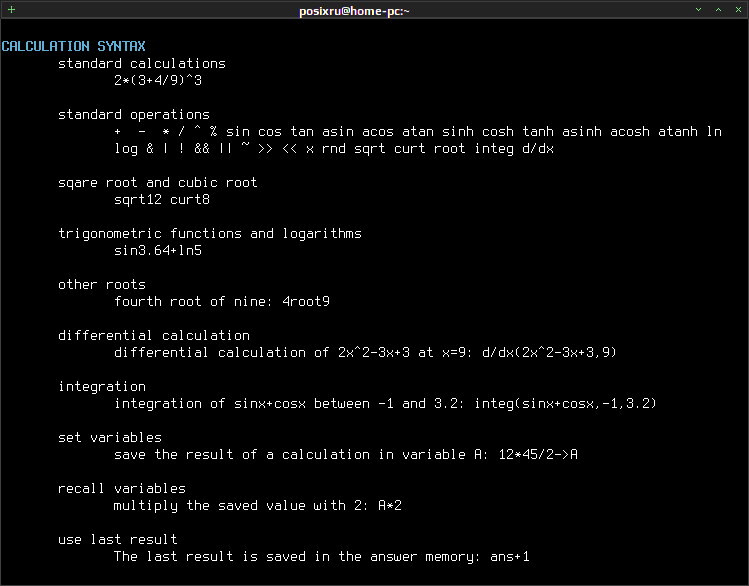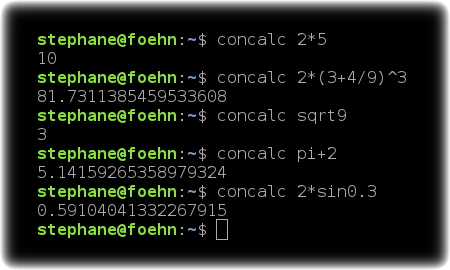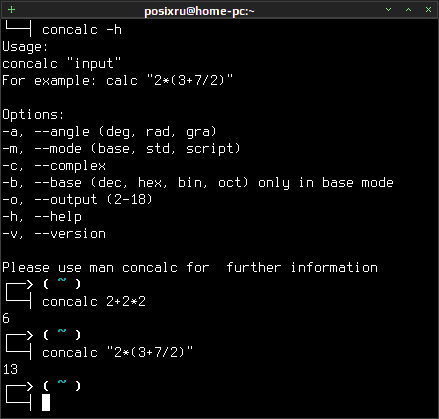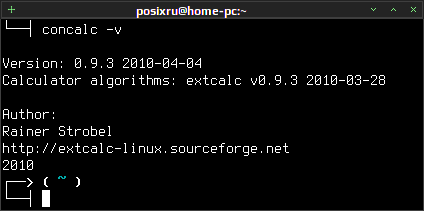# Concalc

concalc — a flexible C++ console calculator for simple and scientific calculations.concalc is a simple wrapper for parsing algorithm Extcalc (parser-algorithm). Using the calculator can handle simple arithmetic and scientific computing.concalc allows you to specify one line with some calculations, the result will be merged, also the calculator can execute scripts written in C (C) like (C-like) programming language.